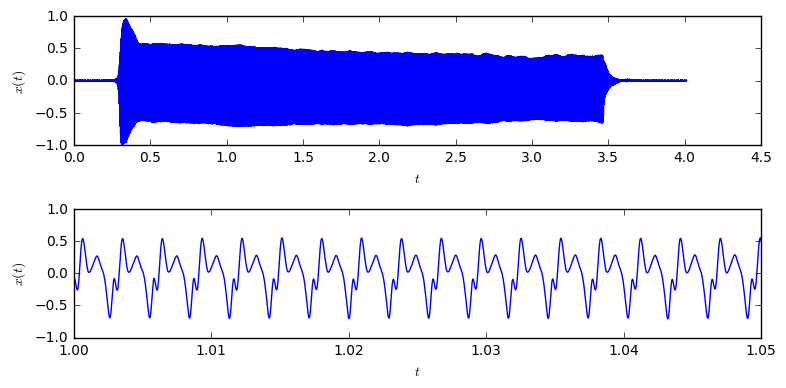# The Sound of Harmonics - Approximating instruments with Fourier Series¶

In a previous article about the Fourier Series calculation we have illustrated how different numbers of harmonics approximate artificial periodic functions. The present post applies the results to the analysis of instrument sounds, namely sounds of a saxophone.

When playing a stationary tone on a saxophone, we hear a constant sound. Hence, we can assume its waveform is periodic, since we could start to listen to the tone at any time and would still hear the same tone. So, the waveform needs to repeat itself over and over again. In this case, it should be possible to expand the waveform into sines and cosines of harmonic frequencies and reconstruct the original signal from them.

We want to verify this is assumption with this post. Let us start with functions to calculate the Fourier series, fourierSeries and for reconstructing a signal from its Fourier series coefficients, reconstruct.

def fourierSeries(period, N):
"""Calculate the Fourier series coefficients up to the Nth harmonic"""
result = []
T = len(period)
t = np.arange(T)
for n in range(N+1):
an = 2/T*(period * np.cos(2*np.pi*n*t/T)).sum()
bn = 2/T*(period * np.sin(2*np.pi*n*t/T)).sum()
result.append((an, bn))
return np.array(result)

def reconstruct(P, anbn):
"""Sum up sines and cosines according to the coefficients to
produce a reconstruction of the original waveform"""
result = 0
t = np.arange(P)
for n, (a, b) in enumerate(anbn):
if n == 0:
a = a/2
result = result + a*np.cos(2*np.pi*n*t/P) + b * np.sin(2*np.pi*n*t/P)
return result


First of all, let's load three sounds of saxophone sounds from the internet:

def loadSound(url):
R = requests.get(url)
data = data * 1.0 / (abs(data).max())
if len(data.shape) > 1:  # for stereo data, use only first channel
data = data[:,0]
return data, rate

# the URLs of the wav files
"http://cd.textfiles.com/sbsw/INSTRMNT/TENORSA.WAV",
sounds = []
for url in urls:
sounds.append((sound, rate))

# Utility function two display two audios side by side in the notebook
def audioSideBySide(name1, audio1, name2, audio2):
text = '%s%s%s%s' % (name1, name2, audio1._repr_html_(), audio2._repr_html_())
display(HTML(text))


for n, s in enumerate(sounds):
display(HTML("Saxophone sound %d" % (n+1) + Audio(data=s, rate=s)._repr_html_()))

Saxophone sound 1
Saxophone sound 2
Saxophone sound 3

They are pretty different, but all clearly sounding like a saxophone. Let's look at the waveform of one sounds to see if its wave is indeed periodic:

t = np.arange(len(sounds)) / sounds
plt.subplot(211)
plt.plot(t, sounds)
plt.subplot(212)
plt.plot(t, sounds)The upper figure shows the whole waveform. There, we can not see any large-scale periodicity. This is clear, since in the original sound we also can hear the start and end of the tone. Looking at the second figure, which shows a zoomed-in version, we can clearly see a very stable periodicity. So, we should be able to extract one period out of this signal and expand it into a Fourier series. Let's do this:

def extractPeriod(data, rate, t_start, t_end):
t = np.arange(0,len(data))/rate
plt.plot(t, data)

duration = t_end - t_start
plt.xlabel('$t$'); plt.ylabel('$x(t)$');

sample_start = int(t_start * rate)
sample_end = int(t_end*rate)

period = data[sample_start:sample_end]
audioSideBySide("Original", Audio(data=data,rate=rate),
"Extracted period", Audio(np.tile(period, int(1/duration)), rate=rate))
return period, rate

periods = []
rates = []
# The manually found start and end time of one period of the signal
periodBounds = [(0.8005,0.8034),
(0.8044,0.8075),
(0.20555,0.2132)]
for n, (S, P) in enumerate(zip(sounds, periodBounds)):
plt.subplot(len(sounds), 1, n+1)
period, rate = extractPeriod(S, S, P, P)
periods.append(period); rates.append(rate)

 Original Extracted period